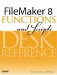# Hour()

Category: Time

 Syntax: Hour ( time )

Parameters:

timeAny valid time value or expression that returns a valid time value.

Data type returned: Number

Description:

The Hour() function returns an integer representing the number of hours specified by the time parameter.

When its parameter represents a specific time of day, the Hour() function returns a value from 0 to 23. To map this into the more familiar 1 to 12 range, you can use the following formula:

``` Mod ( Hour (time) -1; 12 ) + 1
```

The Hour() function can return an integer value outside of the 0 to 23 range when its parameter represents a duration rather than a specific time of day. For instance, Hour ("65:12:53") returns 65.

Examples:

Function

Results

Hour ("10:45:20")

Returns 10.

Hour ("12:15 am")

Returns 0.

Hour ("11:15 pm")

Returns 23.

Hour ( Get(CurrentTime ))

Will return a value from 0 to 23.FileMaker 8 Functions and Scripts Desk Reference
ISBN: 0789735113
EAN: 2147483647
Year: 2004
Pages: 352